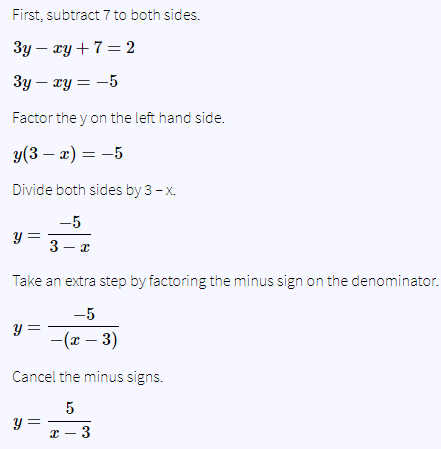# MCQ Functions - Linear and other Functions

The CAT Online Free Test Prep MCQs Functions - Linear and other Functions

### Functions: Linear and other Functions

#### Question:

Solve for y in terms of x.

$3y-xy+7=2$

Option A:      $y=\frac{3}{x-5}$
Option B:      $y=x+\frac{3}{5}$
Option C:      $y=\frac{5}{x-3}$
Option D:      $y=\frac{-5}{x-3}$
Option E:      $y=\frac{5}{x+3}$